• 2019-08-07 10:30:40

## 基本概念

7段S型加减速算法（7 segments S-curve velocity profile），是由T型加速度曲线(Trapezoidal motion profile)演化而来(此外，还有多项式规划（Polynomial Profile），如三次多项式、五次多项式、七次多项式等。虽然五次以上多项式曲线可以让速度加速度都连续变化，但速度曲线没有匀速运动时间，不利于对加减速过程进行优化。)，通过在速度曲线的加速段与匀速段之间增加抛物线(parabolic)或者三角函数(triangular)过度，是的加速度平滑(smooth)过渡，从而解决了加区间两端速度不连续(discontinuous)的问题。顾名思义，典型的7段S型加速度曲线包括如下7段：加加速（increase acceleration）、匀加速(constant acceleration)、减加速（decrease acceleration）、匀速、加减速（increase deceleration）、匀减速（constant deceleration）、减减速（decrease deceleration）。通过指定始末位移量(displacement)，最大允许转速(Vel)、最大允许加速度(acc)、以及加加速度(jerk)，在减少对系统冲击与振动的前提下，充分利用电机伺服性能，以最快的速度（时间最短,）完成运动插补。

## 问题提出

多轴联动必须考虑时间同步(synchronize)，这本质上是一个 non-convex optimization 问题 ，Since there are constraints on initial and final velocities which can be non-zero it is necessary to synchronize each DOF in time 。 Suppose DOF ii takes time TT to reach its destination, you need to synchronize other DOFs with time t=Tt=T, because DOF ii cannot go any faster 。

## 参考资料

### 参考一：

解决方案：velocity profile stretching,we stretch the velocity profile such that it has duration as specified earlier。

#### 链接1

看这篇文章，可能要翻一下.
先分别求出每个独立关节的S曲线，以总规划时间最短（或运动距离最远）的关节时间T为优化对象，再对其他关节进行速度优化调整。

#### 链接2

github.com/rdiankov/openrave.
相比上篇，约束条件少一些，并且给出了实现过程以及源码。
必须指出的是， given a duration tt to synchronize a trajectory with, the problem is not always feasible due to inoperative time interval (参考2有提到). Basically, due to velocity and acceleration limits of the robot joints, given a set of boundary conditions (such as initial and final velocities), there might exists some time interval t = [ t0 , t1 ] such that there exists no trajectory which satisfies the boundary conditions and has a duration t.

#### 链接3

Trajectory Planning for Automatic Machines and Robots.
By Long Smith:I have found a solution. Quiet simple actually. To generate minimum time double s-curve profile I used algorithm presented in the wonderful book above. After computing trajectory for the first DOF I perform the same algorithm for the other DOFs but if trajectory is infeasible with given time, it is appeared to be a good idea to make maximum possible acceleration smaller and smaller with some factor(I use 0.95) which leads to longer trajectory execution. Moreover we can do the same with maximum velocity and jerk constraints which will make more trajectories to be feasible. However this will take significantly more time and since all my tests passed with only decreasing acceleration I am satisfied.

### 参考二

google search key words: s-curve profile synchronize PDF

## 实现方案

对于七段S加减速，这里不再赘述，可以参考一下几篇博文：
CSDN博主–septembernine–S形加减速.
CSDN博主–勤为本–【龙印】步进电机使用七段式抛物线型S曲线加减速和路径规划的理论分析和实现.
这里，主要基于已有的S加减速算法，以及链接2、3(链接2中有S加减速github源码），增加多轴时间约束，达到多轴时间同步的目的。

## 后续

后续会持续跟进，后期会更新处理结果以及相关资源。感兴趣的同学欢迎私我进行沟通。
更新于20210129：鉴于私下很多人联系我讨论多轴同步与指定时间规划的问题，这里补充说明一下：本人近期打算开始对这一块对相关C代码进行测试整理，到时会分享源码，欢迎做机械臂轨迹插补的同行一起交流哈

更多相关内容
• 写在前面 在前面的博文中： 基于抛物线过渡（梯形加减速）的空间直线插补算法与空间圆弧插补算法（Matlab）...下面我尝试了S型加减速曲线的规划方法并结合到空间插补算法中，仿真效果还可以，速度曲线连续，更柔...

## 写在前面

### 学习代码都记录在个人github上，欢迎关注~

Matlab机器人工具箱版本9.10

在前面的博文中：

基于抛物线过渡（梯形加减速）的空间直线插补算法与空间圆弧插补算法（Matlab）

基于单位四元数的姿态插补（Matlab）

我使用了抛物线过渡（也就是梯形加减速）作为空间插补算法的速度规划方法，但是梯形曲线的缺点也很明显，即加速度不连续，速度过渡不平滑，容易造成机械系统冲击。下面我尝试了S型加减速曲线的规划方法并结合到空间插补算法中，仿真效果还可以，加速度曲线连续，更柔和，适用于精度速度要求更高的场合。

空间直线插补：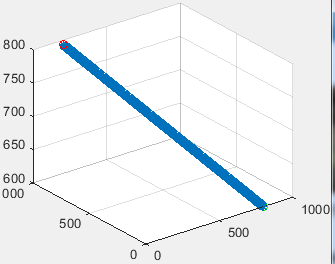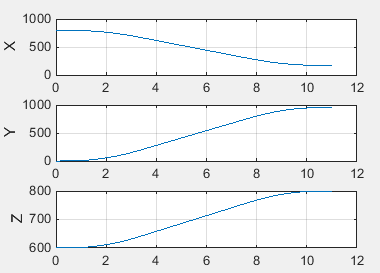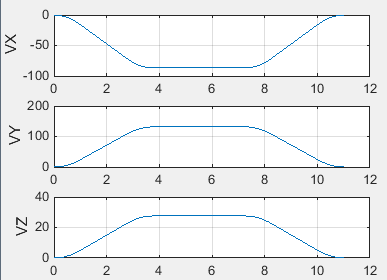空间圆弧插补：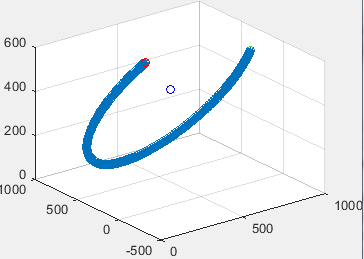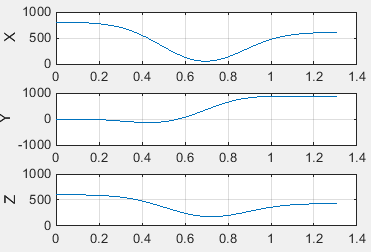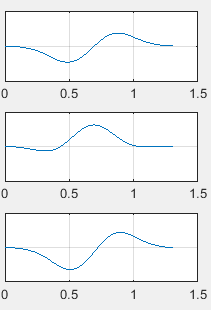直观插补：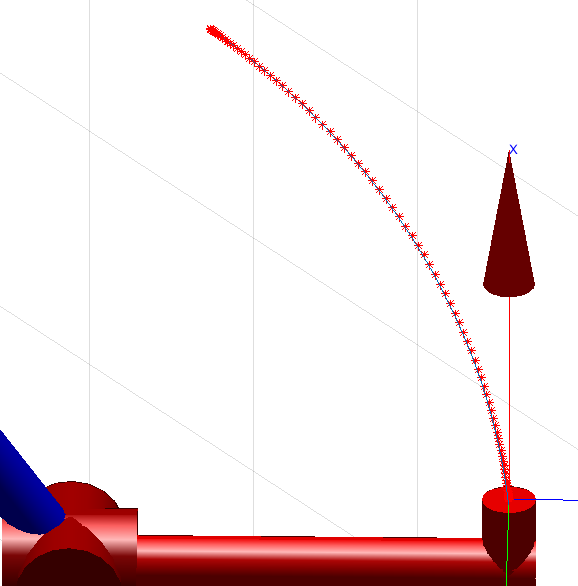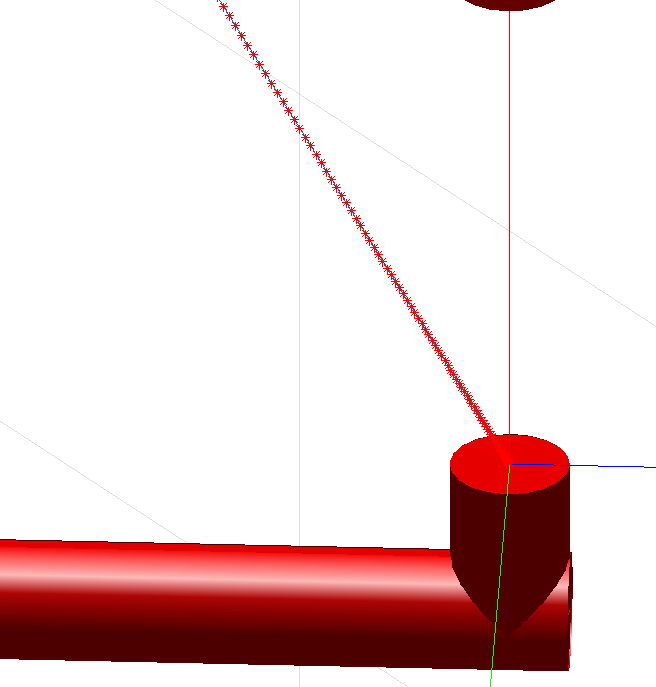## 带约束S型速度规划

预备知识：

机器人学回炉重造（6）：关节空间规划方法——梯形加减速（与抛物线拟合的线性函数）、S型曲线规划

参照基于抛物线过渡（梯形加减速）的空间直线插补算法与空间圆弧插补算法（Matlab）中归一化参数思想，将归一化参数计算函数中的梯形加减速方法换成S型加减速法。此时，定义归一化时间算子：
λ ( t ) = s ( t ) − s ( 0 ) L \lambda( t ) = \frac { s ( t ) - s ( 0 ) } { L }
其中， t ∈ [ 0 , T ] t \in [ 0 , T ] , s ( t ) s(t) 是S型曲线中位移在全局时间坐标下的函数， L L 是机器人执行器末端经过的距离。

## 空间直线插补与空间圆弧插补

位置插补与姿态插补需要分开讨论。

### 位置插补

假设机器人末端由P1点沿直线运动到P2点， ( x 1 , y 1 , z 1 ) \left(x_{1}, y_{1}, z_{1}\right) 分别为起点P1的位置， ( x 2 , y 2 , z 2 ) \left(x_{2}, y_{2}, z_{2}\right) 为终点P2的位置， ( x i , y i , z i ) \left(x_{i}, y_{i}, z_{i}\right) 为中间插补点Pi的位置。

各个插值点位置坐标向量为：
{ x = x 1 + λ Δ x y = y 1 + λ Δ y z = z 1 + λ Δ z \left\{\begin{array}{l}{x=x_{1}+\lambda \Delta x} \\ {y=y_{1}+\lambda \Delta y} \\ {z=z_{1}+\lambda \Delta z}\end{array}\right.
其中， λ \lambda 是归一化时间因子， ( Δ x , Δ y , Δ z ) (\Delta x, \Delta y, \Delta z) 表示首末位置间的位置增量，其解为：
{ Δ x = x 2 − x 1 Δ y = y 2 − y 1 Δ z = z 2 − z 1 \left\{\begin{array}{l}{\Delta x=x_{2}-x_{1}} \\ {\Delta y=y_{2}-y_{1}} \\ {\Delta z=z_{2}-z_{1}} \end{array}\right.

### 基于单位四元数的姿态插补

预备知识：

基于单位四元数的姿态插补（Matlab）

已知起点S、终点D的齐次变换矩阵（位姿）分别为 T S T_S T D T_D ，则姿态插补步骤如下：

1. 从齐次变换矩阵中提取出各自的旋转矩阵 R S R_S R D R_D ，并将其转换为四元数 Q s Q_s Q d Q_d ;

2. 姿态四元数插补公式为：
Q k ( t ) = Slerp ⁡ ( Q s , Q d , t ) = sin ⁡ [ ( 1 − λ ( t ) ) θ ] sin ⁡ θ Q s + sin ⁡ [ λ ( t ) θ ] sin ⁡ θ Q d Q_{k}(t)=\operatorname{Slerp}\left(Q_{s}, Q_{d}, t\right)=\frac{\sin [(1-\lambda(t)) \theta]}{\sin \theta} Q_{s}+\frac{\sin [\lambda(t) \theta]}{\sin \theta} Q_{d}
式中， θ = a r c c o s ( Q s ⋅ Q d ) \theta=arccos(Q_{s} \cdot Q_{d})

3. 将四元数 Q k ( t ) Q_{k}(t) 转换为旋转矩阵 R k R_{k} ，将 R k R_{k} 和位置插补得到的 P k P_{k} 组合得到各插值点对应的齐次变换矩阵 T k T_{k}

4. 带入逆运动学计算，得到各关节角度变量，进行插补运动。

## 规划插补流程

基于带约束S型加减速曲线的插补算法流程图如下：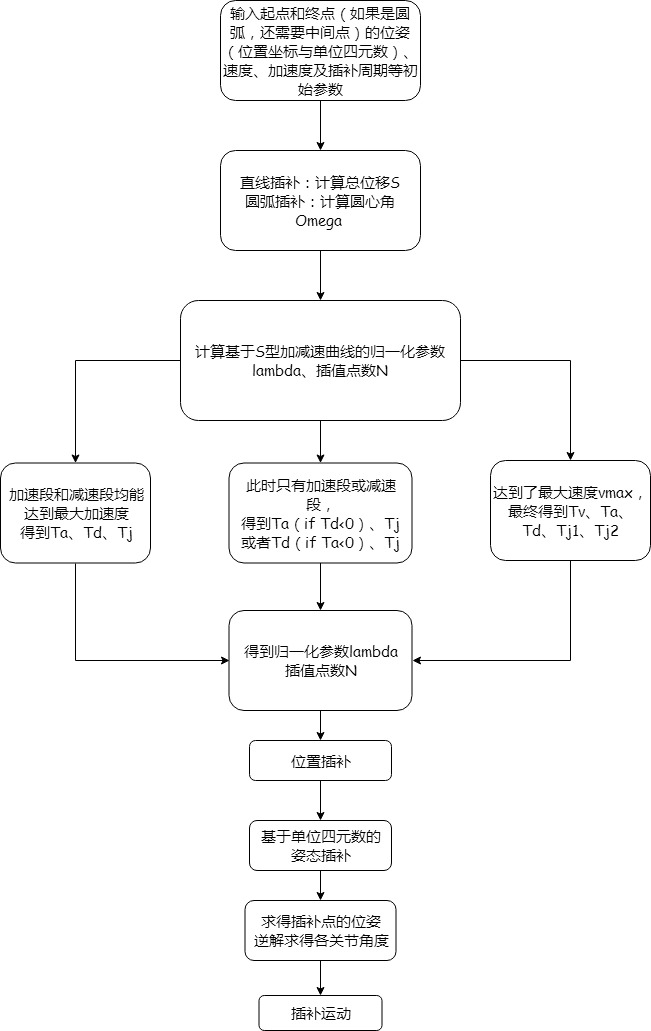## Matlab实现代码

和前面博文的联系非常紧密，部分函数并未展出。

% 归一化处理
% S型加减速曲线
% 输入参数：机械臂末端运动总位移（角度）pos
%          机械臂末端线速度（角速度）初始v0，终止v1，最大速度vmax
%          最大加减速度amax、最大加加速度jmax
%          插补周期t
% 返回值： 插值点数N
function [lambda, N] = Normalization_S(pos, v0, v1, vmax, amax, jmax, t)
% S曲线参数计算（S型速度规划，又称七段式轨迹）
% function para = STrajectoryPara(q0, q1, v0, v1, vmax, amax, jmax)
q0 = 0; q1 = pos;
para = STrajectoryPara(q0, q1, v0, v1, vmax, amax, jmax); % 获取S曲线参数
% 总的规划时间
T = para(1) + para(2) + para(3)
% 等时插补
N = ceil(T / t);
j = 1;
for i = 0 : t: T
q(j) = S_position(i, para(1), para(2), para(3), para(4), para(5), para(6), para(7), para(8), para(9), para(10), para(11), para(12), para(13), para(14), para(15), para(16));
%    qd(j) = S_velocity(i, para(1), para(2), para(3), para(4), para(5), para(6), para(7), para(8), para(9), para(10), para(11), para(12), para(13), para(14), para(15), para(16));
lambda(j) = q(j) / pos;
j = j + 1;
end
end

% 空间单一直线位置插补与单元四元数姿态插补 + 梯形加减速归一化处理/S型加减速曲线归一化处理
% 参数：起点S位置， 终点D位置
%      起点S的单元四元数Qs，终点的单元四元数Qd
%      起始速度v0，终止速度v1，最大速度vmax，最大加速度amax，最大加加速度jmax
%      插补周期t
% 返回值：插值点位置、单元四元数、插值点数
function [x y z qk N] = SpaceLine_Q(S, D, Qs, Qd, v0, v1, vmax, amax, jmax, t)
x1 = S(1); y1 = S(2); z1 = S(3);
x2 = D(1); y2 = D(2); z2 = D(3);

P = 1; % 插值参数，增加插值点数，避免过小
% 总位移S
s = sqrt(power(x2 - x1, 2) + power(y2 - y1, 2) + power(z2 - z1, 2))
% 插值点数N
% N = ceil(P*s / vs)
% 求归一化参数：梯形加减速曲线
% function lambda = Normalization(pos, vel, accl, N)
% lambda = Normalization(s, vs, a, N);
% 求归一化参数：S型加减速曲线
% function lambda = Normalization_S(pos, v0, v1, vmax, amax, jmax, N)
[lambda, N] = Normalization_S(s, v0, v1, vmax, amax, jmax, t);
delta_x = x2 - x1;
delta_y = y2 - y1;
delta_z = z2 - z1;
% 计算两个四元数之间的夹角
dot_q = Qs.s*Qd.s + Qs.v(1)*Qd.v(1) + Qs.v(2)*Qd.v(2) + Qs.v(3)*Qd.v(3);
if (dot_q < 0)
dot_q = -dot_q;
end

for i = 1: N
% 位置插补
x(i) = x1 + delta_x*lambda(i);
y(i) = y1 + delta_y*lambda(i);
z(i) = z1 + delta_z*lambda(i);
% 单位四元数球面线性姿态插补
% 插值点四元数
if (dot_q > 0.9995)
k0 = 1-lambda(i);
k1 = lambda(i);
else
sin_t = sqrt(1 - power(dot_q, 2));
omega = atan2(sin_t, dot_q);
k0 = sin((1-lambda(i)*omega)) / sin(omega);
k1 = sin(lambda(i)*omega) / sin(omega);
end
qk(i) = Qs * k0 + Qd * k1;
end

end

% 空间单一圆弧位置插补与单位四元数姿态插补 + 梯形加减速归一化处理/S型加减速曲线归一化处理
% 参数： 起点S位置, 中间点M位置, 终点D位置
%       起点S和终点D的单位四元数Qs_,Qd_
%       起始速度v0，终止速度v1，最大速度vmax，最大加速度amax，最大加加速度jmax
%       插值周期t
% 返回值：插值点位置、单元四元数、插值点数
% 方便起见，角速度和角加速度均为角度制
function [x y z qk N] = SpaceCircle_Q(S, M, D, Qs_, Qd_, v0, v1, vmax, amax, jmax, t)
x1 = S(1); x2 = M(1); x3 = D(1);
y1 = S(2); y2 = M(2); y3 = D(2);
z1 = S(3); z2 = M(3); z3 = D(3);

A1 = (y1 - y3)*(z2 - z3) - (y2 - y3)*(z1 - z3);
B1 = (x2 - x3)*(z1 - z3) - (x1 - x3)*(z2 - z3);
C1 = (x1 - x3)*(y2 - y3) - (x2 - x3)*(y1 - y3);
D1 = -(A1*x3 + B1*y3 + C1*z3);

A2 = x2 - x1;
B2 = y2 - y1;
C2 = z2 - z1;
D2 = -((x2^2 - x1^2) + (y2^2 - y1^2) + (z2^2 - z1^2)) / 2;

A3 = x3 - x2;
B3 = y3 - y2;
C3 = z3 - z2;
D3 = -((x3^2 - x2^2) + (y3^2 - y2^2) + (z3^2 - z2^2)) / 2;
A = [A1, B1, C1; A2, B2, C2; A3, B3, C3]
b = [-D1, -D2, -D3]'
% 圆心
C = Gauss_lie(3, A, b)
x0 = C(1); y0 = C(2); z0 = C(3);
plot3(x0, y0, z0, 'bo')
hold on
% 外接圆半径
r = sqrt(power(x1 - x0, 2) + power(y1 - y0, 2) + power(z1 - z0, 2));
% 新坐标系Z0的方向余弦
L = sqrt(A1^2 + B1^2 + C1^2);
ax = A1 / L; ay = B1 / L; az = C1 / L;
% 新坐标系X0的方向余弦
nx = (x1 - x0) / r;
ny = (y1 - y0) / r;
nz = (z1 - z0) / r;
% 新坐标系Y0的方向余弦
o = cross([ax, ay, az], [nx, ny, nz]);
ox = o(1);
oy = o(2);
oz = o(3);
% 相对于基座标系{O-XYZ}， 新坐标系{C-X0Y0Z0}的坐标变换矩阵
T = [nx ox ax x0;
ny oy ay y0;
nz oz az z0;
0  0  0  1]
T_ni = T^-1
% 求在新坐标系{C-X0Y0Z0}下S、M和D的坐标
S_ = (T^-1)*[S'; 1]
M_ = (T^-1)*[M'; 1]
D_ = (T^-1)*[D'; 1]
x1_ = S_(1) , y1_ = S_(2), z1_ = S_(3)
x2_ = M_(1), y2_ = M_(2), z2_ = M_(3)
x3_ = D_(1), y3_ = D_(2), z3_ = D_(3)
% 判断圆弧是顺时针还是逆时针，并求解圆心角
if (atan2(y2_, x2_) < 0)
angle_SOM = atan2(y2_, x2_) + 2*pi;
else
angle_SOM = atan2(y2_, x2_);
end
if (atan2(y3_, x3_) < 0)
angle_SOD = atan2(y3_, x3_) + 2*pi;
else
angle_SOD = atan2(y3_, x3_);
end
% 逆时针
if (angle_SOM < angle_SOD)
flag = 1;
theta = angle_SOD % 圆心角
end
% 顺时针
if (angle_SOM >= angle_SOD)
flag = -1;
theta = 2*pi - angle_SOD % 圆心角
end
% 插补点数N
% P = 1; %插补参数，增加插值点数，避免过小
% ws = ws*pi / 180; % 角度换成弧度
% a = a*pi / 180;
% N = ceil(P*theta / ws);
% % 求归一化参数：梯形加减速曲线
% lambda = Normalization(theta, ws, a, N);
% 求归一化参数：S型加减速曲线
% function lambda = Normalization_S(pos, v0, v1, vmax, amax, jmax, N)
[lambda, N] = Normalization_S(theta, v0, v1, vmax, amax, jmax, t);

% 插补原理: 在新平面上进行插补（简化）
% 在新坐标系下z1_,z2_,z3_均为0，即外接圆在新坐标系的XOY平面内
% 此时转化为平面圆弧插补问题
delta_ang = theta;
% 计算两个四元数之间的夹角
dot_q = Qs_.s*Qd_.s + Qs_.v(1)*Qd_.v(1) + Qs_.v(2)*Qd_.v(2) + Qs_.v(3)*Qd_.v(3);
if (dot_q < 0)
dot_q = -dot_q;
end

for i = 1: N
% 位置插补
x_(i) = flag * r * cos(lambda(i)*delta_ang);
y_(i) = flag * r * sin(lambda(i)*delta_ang);
P = T*[x_(i); y_(i); 0; 1];
x(i) = P(1);
y(i) = P(2);
z(i) = P(3);
% 单位四元数球面线性姿态插补
% 插值点四元数
if (dot_q > 0.9995)
k0 = 1-lambda(i);
k1 = lambda(i);
else
sin_t = sqrt(1 - power(dot_q, 2));
omega = atan2(sin_t, dot_q);
k0 = sin((1-lambda(i))*omega) / sin(omega);
k1 = sin(lambda(i)*omega) / sin(omega);
end
qk(i) = Qs_ * k0 + Qd_ * k1;
end

end


## 不足之处

1. 圆弧的姿态插补只严格通过了起始姿态和终点姿态，没有经过中间姿态；
2. 无法达到时间最优。

## 参考文献

刘鹏飞,杨孟兴,宋科,段晓妮.‘S’型加减速曲线在机器人轨迹插补算法中的应用研究[J].制造业自动化,2012,34(20):4-8+11.

李振娜,王涛,王斌锐,郭振武,陈迪剑.基于带约束S型速度曲线的机械手笛卡尔空间轨迹规划[J].智能系统学报,2019,14(04):655-661.

王添. 基于单位四元数机器人多姿态轨迹平滑规划[D].天津工业大学,2018.

季晨. 工业机器人姿态规划及轨迹优化研究[D].哈尔滨工业大学,2013.

展开全文• S”形加减速就是指速度曲线中加减速阶段的曲线呈现一个英文字母“S。 “S”形加减速的曲线并没有限定是什么曲线，所以可以是指数曲线，可以是正弦曲线，这里分析的“S”形加减速是基于速度匀速变化的曲线。 ...

# 步进电机S形加减速简介

一个物体从起点运动到终点要经历加速、匀速、减速的过程，把整个过程中速度随时间变化关系画出来，就是速度曲线。
“S”形加减速就是指速度曲线中加减速阶段的曲线呈现一个英文字母“S”型。
“S”形加减速的曲线并没有限定是什么曲线，所以可以是指数曲线，可以是正弦曲线，这里分析的“S”形加减速是基于加速度匀速变化的曲线。
基于加速度匀速变化的S形加减速可分为：
7段：加加速段（T1）、匀加速段（T2）、减加速段（T3）、匀速段（T4）、加减速段（T5）、匀减速段（T6）、减减速度段（T7）。
6段：加加速段（T1）、匀加速段（T2）、减加速段（T3）、加减速段（T5）、匀减速段（T6）、减减速度段（T7）。
5段：加加速段（T1）、减加速段（T3）、匀速段（T4）、加减速段（T5）、减减速度段（T7）。
4段：加加速段（T1）、减加速段（T3）、加减速段（T5）、减减速度段（T7）。

# 七段S形加减速算法原理分析

加速/减速开始时速度比较缓慢，然后逐渐加快，在加速/减速接近结束时速度再次缓慢下来，从而使移动较为稳定。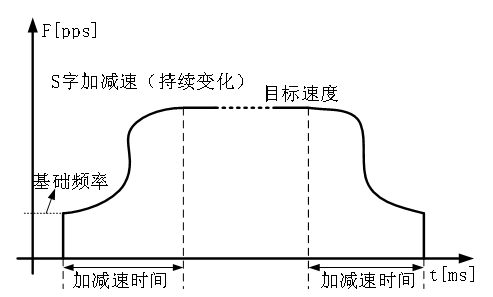“S”形曲线加减速将传统的3段加减速过程变为7段加减速过程，形成S字形。“S”形曲线由加速段（T1）、匀加速段（T2）、减加速段（T3）、匀速段（T4）、减速段由加减速段（T5）、匀减速段（T6）、减减速度段（T7）组成。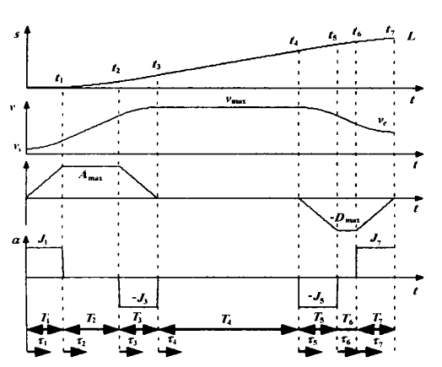位移，速度，加速度，加加速度如图所示。
设初速度为Vs，末速度为Ve，最大速度为Vmax。加加速度J记为Jmax。根据S曲线对称性，当可以达到最大速度时，其中T1=T3，T5=T7。
tk(k=0,1,…7) ：表示各个阶段的过渡点时刻，虚线对应的时刻；
Tk(k=1,…7)：各个阶段的持续运行时间。
τk(k=0,1,…7)：局部时间坐标，表示以各个阶段的起始点作为时间零点的时间表示，τk表示以第K段某一时刻与该段起点时刻的时间差。这样以τk作为每一段的自变量，以上一段结束时的速度作为本段的初速度，每一小段单独计算速度值可以大大简化速度表达式，分段计算的思想可以为计算和程序设计带来很大方便。相当于每个段都把竖轴移到虚线处。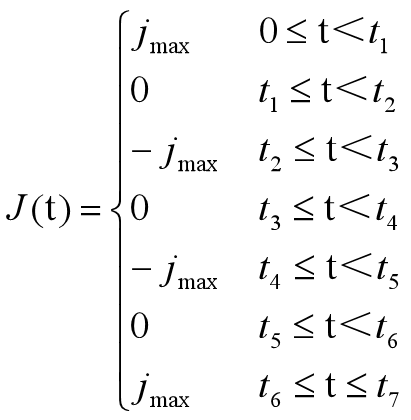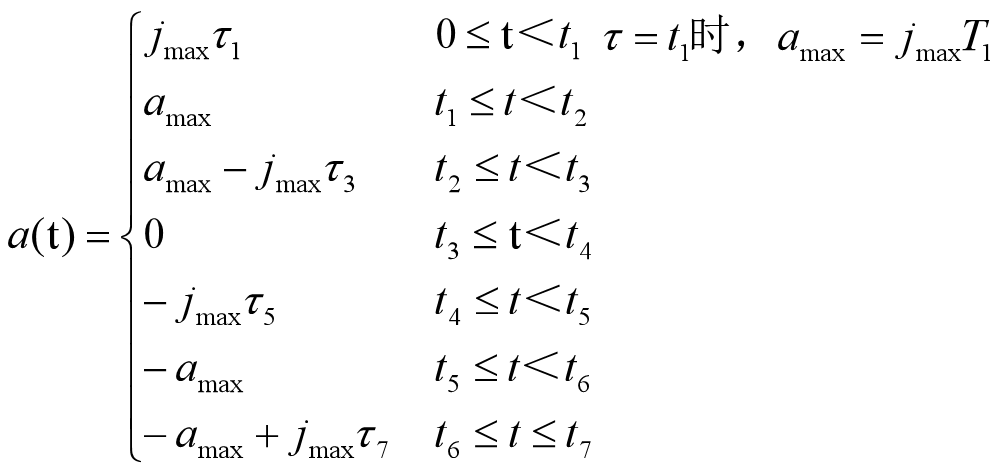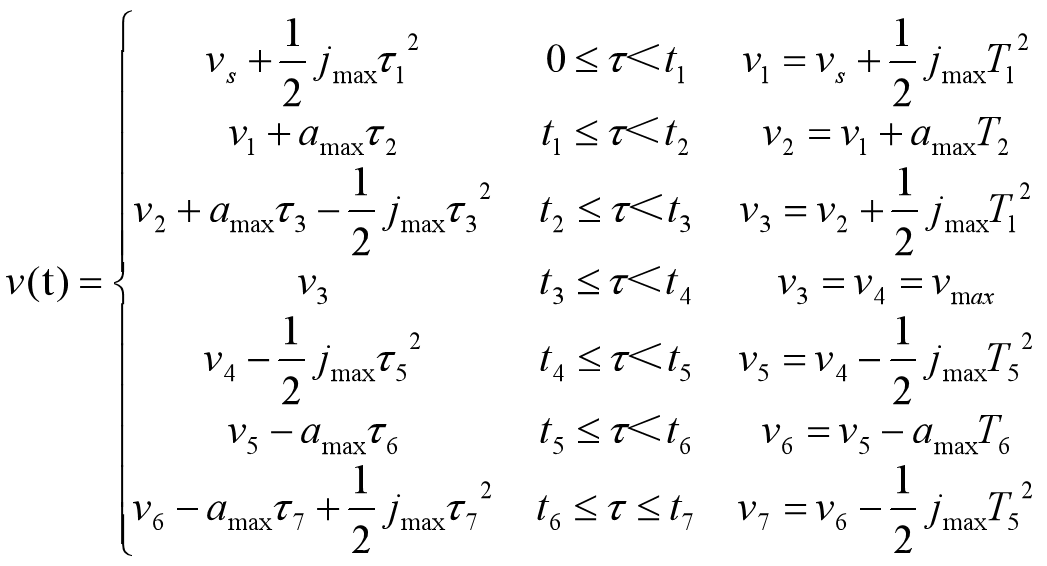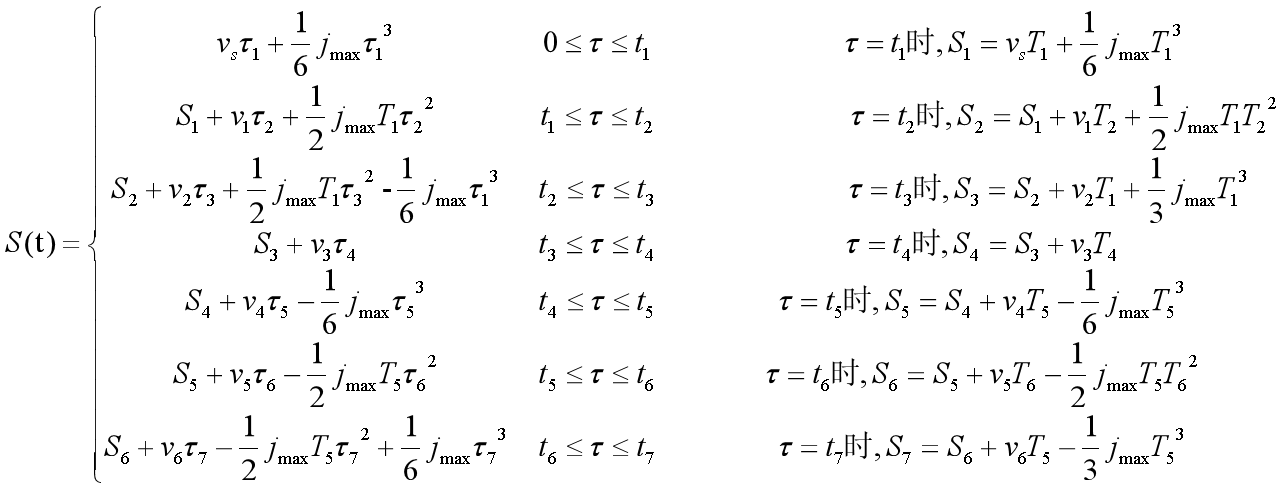传统的七段S曲线是一个完整段数学模型，但在实际应用中S曲线是否存在匀速段取决于速度达到给定速度时，加速段和减速段位移之和是否小于实际需要运行段位移。加速及减速过程中是否存在匀加速段或匀减速段则由步进电机段初始速度Vs、最终速度Ve、加速度amax和加加速度J共同决定。
由S曲线特性可知，加加速段和减加速段对称。且在加速过程中，应尽量满足使加速度a可达到amax。所以，匀加速段存在段条件是给定速度V和初速度Vs之差不小于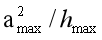1）若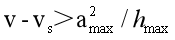，则加速过程中存在匀加速段，最大加速度能够达到amax，加速过程中的加加速段时间、匀加速段时间、减加速段时间为：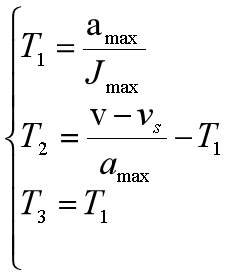2）若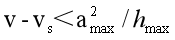，则加速过程中不存在匀加速段，最大加速度不能够达到amax，加速过程中的加加速段时间、匀加速段时间、减加速段时间为：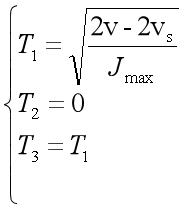由于S曲线的减速段跟加速段对称，按照同样的思路可得出减速阶段加减速时间，匀减速时间和减减速时间为：
1）在的情况下2）在，的情况下：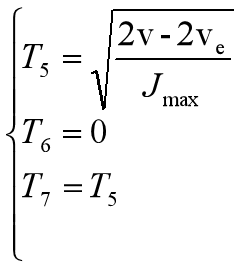还剩下匀速段时间未求：
用SAcc表示步进电机在加速阶段的位移、SDec表示减速段的位移，S表示实际需要运行的位移，则：
1）当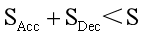时，S曲线中存在匀速运行的阶段，此时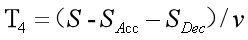2）当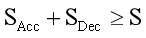时，S曲线中不存在匀速运行的阶段，此时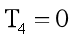至此，全部参数以及确定，在应用时可以根据实际情况规划需要的S曲线。
几个中间计算式推导：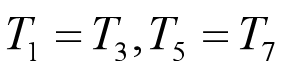加加速度为J保持不变，加速度最大值为amax，那么时间T1为：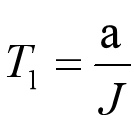加速度曲线为梯形，梯形面积为速度最大值Vmax，初试速度为Vs，可得
匀加速段时间T2：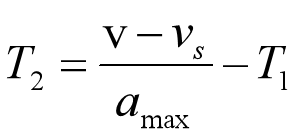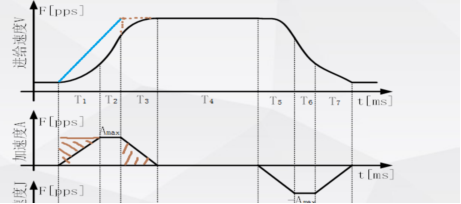割补一下面积，相当于变成了T型加减速。
T4的另一种推法：
匀速段时间T4的大小与位移S有关，如果加速段曲线和减速段曲线对称。
设加速段（1,2,3段对应的加加速，匀加速，减加速）位移之和为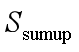那么减速段位移之和也是， 可得：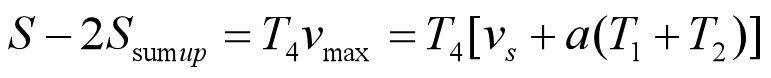根据对称性，在下图中的线段AB与S曲线围城的两个区域面积相等，那么梯形OABC的面积就等于加速段的位移，所以：
梯形面积公式，1/2上底加下底再乘高，其中T1=T3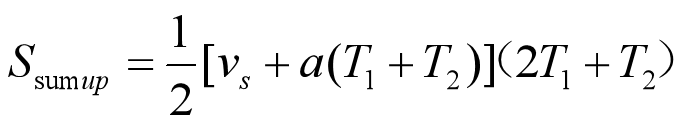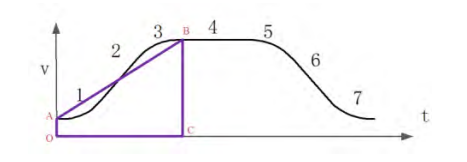将上式带入上上式，可得匀速段持续时间T4：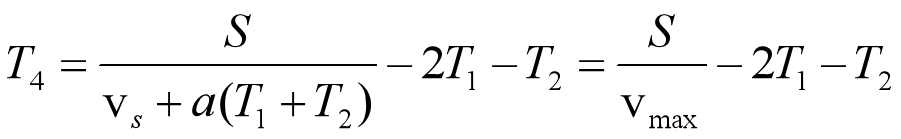# 五段S形加减速算法实现

假设给定条件：加速段的末速度，初速度，加速时间，以此来计算控制步进电机的每一步速度。
加速段由加加速段(IncAccel)和减加速段(DeAccel)组成。
因为T1=T2，T4=T5，为了简化算法初速度V0和最终速度Ve都为0，使T1=T2=T4=T5=T，那么T和T3确定了加加速度，加速度，速度，位移之间的关系就确定了。
加加速段是加速度关于时间的斜率为正的直线，减加速段则是加速度关于时间的斜率为负的直线。
加速度从0开始变化，到了一定值开始减小，最后为0，由于加速度的斜率是相同的，所以加加速度段和减加速度段，所用的时间是一样。这两段曲线是关于加速度最大值的对应的速度中心对称的。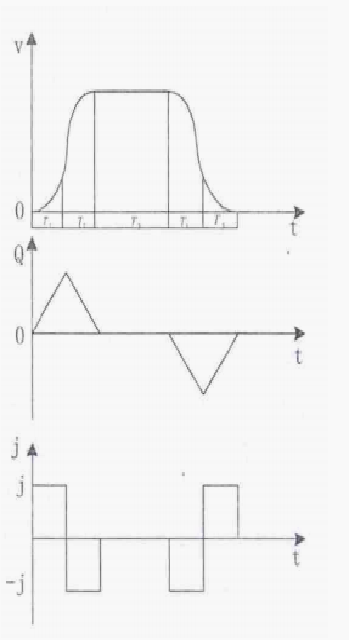针对加加速段分析：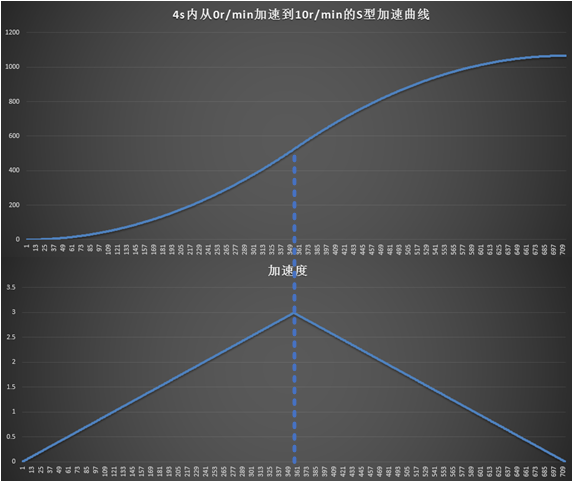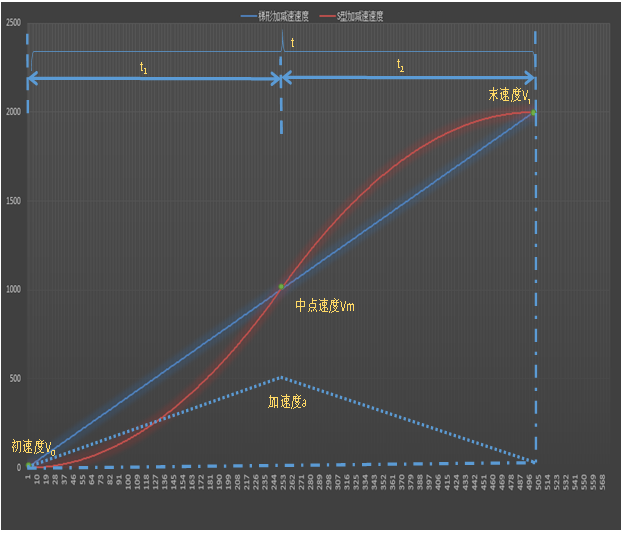# 算法分析

准备工作（推导方法和前面一致）：
针对加加速段分析，此时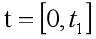，且初速度V0=0：
当加速度从0变化到最大值，有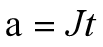J为加加速度（Jerk），对加速度积分面积就是速度，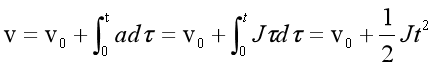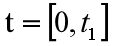速度的变化量：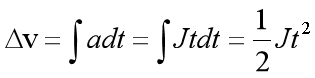，那么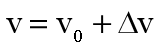只要知道J即可得到加加速段的速度变化，当加速度变化到最大值时，此时
速度（V）=中点速度(VM)，可得：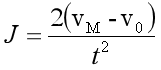其中：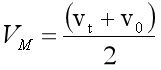vt为末速度，是减加速段最终的速度值，而这一段的距离可以求得：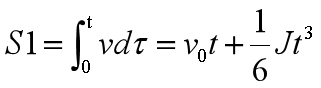针对减加速段分析，此时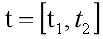，且此阶段初速度为上个阶段的末速度v，此阶段末速度为vt。
当加速度从最大值变化到0，有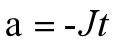J为加加速度（Jerk），对加速度积分面积就是速度，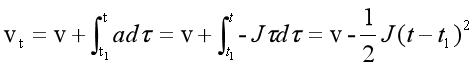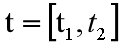速度的变化量：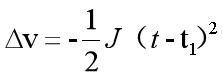那么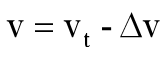，整理成此步是为了方便建立速度表。

而这一段的距离可以求得（平均速度乘时间减去加加速段位移）：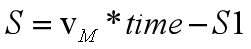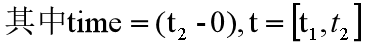整理一下可得：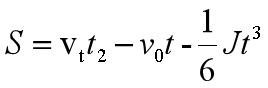对速度而言，只要t变化足够小，即dt小，那么速度变化就越平滑，得到的速度点拟合出来的曲线就越接近目标曲线。
根据以上速度公式只要给定一个合适的时间t，比如在2s内加速了1000步，即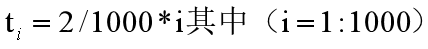，那么t1为第一步的时间，t2为第一步和第二步的时间……（离散时间的方法）
步进电机速度单位为r/min时，可以把单位转换为step/s，方法为：假设速度为5r/min，5细分数（电机转动一圈脉冲数）/60。 其中60为60秒，将r/min转换成了step/s。
同时，步进电机是1个脉冲走1步并旋转一定角度，当电机速度单位为step/s时，速度就等于脉冲间隔频率，即speed=Freq。因为脉冲间隔频率单位为hz，Ahz即1秒产生A个脉冲，可以驱动电机走A步，速度单位为step/s，Astep/s也即1秒电机走A步。

# 查表法编程实现思路及方法

使用查表法如何实现：
对加速段建立速度表，速度单位为step/s时，速度表即为频率表。频率的倒数为时间，C*tt=时间，其中C为定时器计数值，tt为定时器计数周期。最终需要落实到定时器计数值。想让速度曲线为S曲线，在加加速段，频率（速度）的增量由小变大，C由大变小，在减加速段，频率（速度）的增量由大变小，C由小变大。
对减速段则将速度表逆序即可实现减速。
同样的在定时器中断里，设置状态标志：加速，减速，匀速，停止以实现功能。

1.根据已知的速度条件（初速度，末速度）和加速时间，可以求得加加速度J，根据位移公式：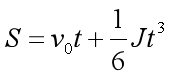可以求得加加速需要的步数，那么减加速步数=平均速度乘时间-加加速步数也可求得。
2.初始化一个速度表用于存速度，注意速度的单位应当转化为step/s。

3.根据位移公式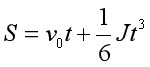，计算走一步的时间，即S=1，此时V0,J,S都已知，
可算出t，记为ti。这个ti就是前面前面提到的离散时间的思想，t1为第一步的时间，t2为第一步和第二步的时间，t2=2*t1……
使用一个简单的for循环来描述：
for（i=1;i<加加速步数;i++）
{
Sumt += Ti; //这样就能计算出1到n步的时间
}
for（i=1;i<加加速步数;i++）
{
Sumt += Ti;
DeltaV = 0.5f * Jerk * pow(Sumt,2);
Speed.VelocityTab[i] = Speed.Vo + DeltaV;
}
这样就计算出了速度的增量，并建立出了加加速阶段的速度表。
值得注意的是当最后一步的时候,时间并不严格等于加加速度时间，还需要处理。

可以在最后一步的前一步不采用累加时间的方式，而是
Sumt = fabs(Sumt - 加加速段时间 )这样来减小误差。
其中DeltaV = 0.5f * Jerk * pow(Sumt,2);Speed.VelocityTab[i] = Speed.Vo + DeltaV;同理对于减加速阶段，建立速度表：

DeltaV = 0.5f * Jerk * pow(fabs( Time - Sumt),2)Speed.VelocityTab[i] = Speed.Vt - DeltaV;至此，整个加速段的速度表建立完毕，而减速段速度表只需要将表倒序。
速度表最终需要落实到定时器计数值上：因为速度表等于脉冲频率表，频率的倒数为时间δt，而C*tt=δt，其中C为定时器计数值，tt为定时器计数周期。
有了这个C值，即可产生PWM波。

4.如何产生PWM波及TIMx定时器配置
使用定时器来产生PWM波：定时器计数器，初始输出低电平，计数小于num值时，输出高电平，大于num值小于2*num值时，输出低电平。这样就产生了一个脉冲。
定时器配置模式可以选择输出比较模式，使用此模式输出PWM波，简单来说就是当计数器与捕获/比较寄存器当前值匹配时，输出比较功能做出不同的动作。比如：当计数器比捕获/比较寄存当前值小时，输出高电平，达到比较值（捕获/比较寄存器当前值）后，翻转电平输出低电平。再重新设置新的比较值，如此反复，即可输出PWM波。

# S曲线加减速流程图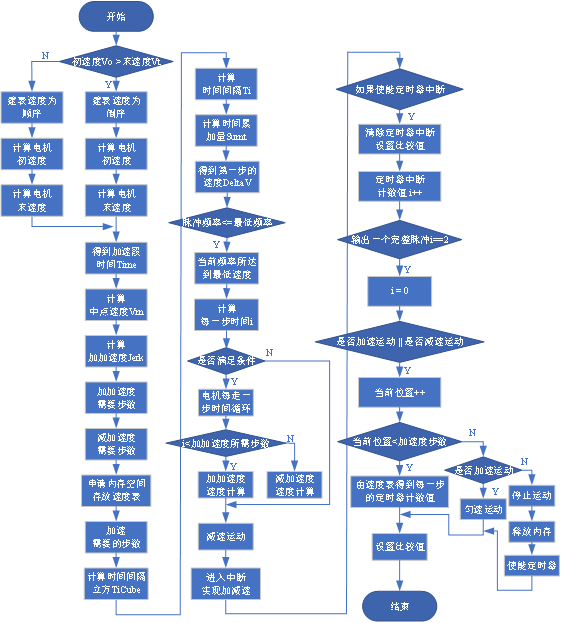注意事项：
“S”形加减速算法设计通过初速度、末速度、中点速度等参数来进行对算法的编写。如果要进行加减速控制首先要建立速度表，建立表时需注意初速度比末速度大时做减速运动，数值变化跟加减运动相同，然后判断建表时速度是倒序还是顺序。建立表最重要的一点就是建表时需申请内存空间存放速度表（malloc），申请内存空间之后一定要记得释放空间（free）。
当建立速度表之后首先要计算第一步速度，根据第一步的速度值达到下一步的时间，步进电机的速度就是定时器脉冲输出频率，即可得到计算每一步的时间。
“S”形做加减速运动时，步进电机如果为运行状态，第一步的速度是0，则定时器从0xFFFF开始，定时器中断服务函数实现加减速运动。中断服务函数通过速度表得到每一步的定时器计数值，然后运动结束之后要释放内存。

展开全文• 写在前面 机械臂用的是五自由度的，我测试时发现逆解精度存在...常规插补算法 假设机器人末端由P1点沿直线运动到P2点，(x1,y1,z1)\left(x_{1}, y_{1}, z_{1}\right)(x1​,y1​,z1​)和(α(1,β1,γ1)\left(\alpha(_{...

## 写在前面

### 学习代码都记录在个人github上，欢迎关注~

Matlab机器人工具箱版本9.10

机械臂用的是五自由度的，我测试时发现逆解精度存在一些问题，目前还没找到是求解析解时出错还是编程过程有问题，还是算法本身考虑不周到，欢迎有研究过的大神们批评指正！感谢~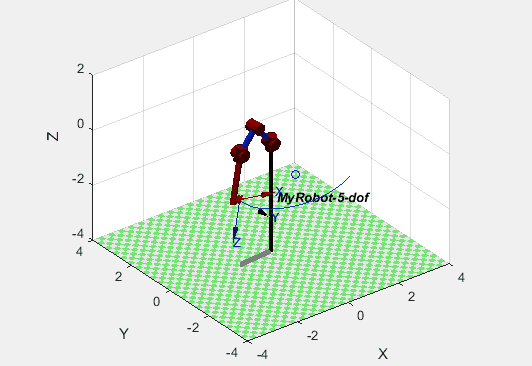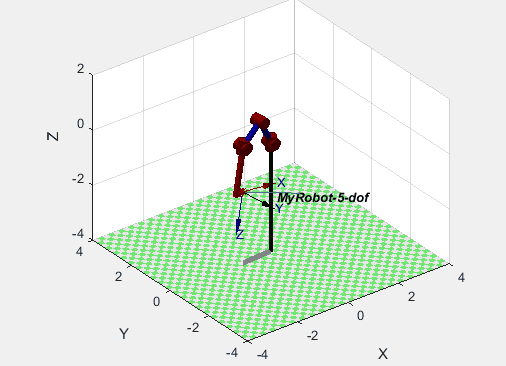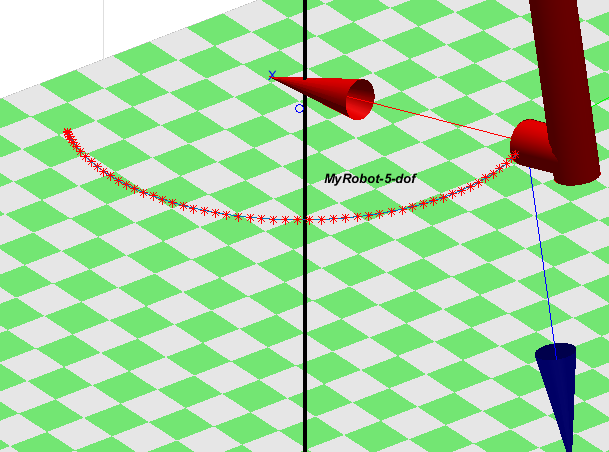## 常规插补算法

假设机器人末端由P1点沿直线运动到P2点， ( x 1 , y 1 , z 1 ) \left(x_{1}, y_{1}, z_{1}\right) ( α ( 1 , β 1 , γ 1 ) \left(\alpha(_{1}, \beta_{1}, \gamma_{1}\right) 分别为起点P1的位置和RPY角， ( x 2 , y 2 , z 2 ) \left(x_{2}, y_{2}, z_{2}\right) ( α 2 , β 2 , γ 2 ) \left(\alpha_{2}, \beta_{2}, \gamma_{2}\right) 分别为终点P2的位置和RPY角， ( x i , y i , z i ) \left(x_{i}, y_{i}, z_{i}\right) ( α i , β i , γ i ) \left(\alpha_{i}, \beta_{i}, \gamma_{i}\right) 分别为中间插补点Pi的位置和RPY角。

各个插值点位置坐标向量和RPY角度向量可以表示为：
{ x = x 1 + λ Δ x y = y 1 + λ Δ y z = z 1 + λ Δ z （ 1 ） \left\{\begin{array}{l}{x=x_{1}+\lambda \Delta x} \\ {y=y_{1}+\lambda \Delta y} \\ {z=z_{1}+\lambda \Delta z}\end{array}\right.（1）

{ α = α 1 + λ Δ α β = β 1 + λ Δ β γ = γ 1 + λ Δ γ （ 2 ） \left\{\begin{array}{l}{\alpha=\alpha_{1}+\lambda \Delta \alpha} \\ {\beta=\beta_{1}+\lambda \Delta \beta} \\ {\gamma=\gamma_{1}+\lambda \Delta \gamma}\end{array}\right.（2）

其中， ( x , y , z ) (x, y, z) ( α , β , γ ) (\alpha, \beta, \gamma) 表示插值点的位置坐标向量和RPY角度向量， λ \lambda 为归一化因子，采用抛物线过渡的线性函数（即梯形加减速）以保证整段轨迹上的位移和速度都连续。 ( Δ x , Δ y , Δ z ) (\Delta x, \Delta y, \Delta z) ( Δ α , Δ β , Δ γ ) (\Delta \alpha, \Delta \beta, \Delta \gamma) 分别表示首末位置间的位置和RPY角度的增量，其求解为：
{ Δ x = x 2 − x 1 Δ y = y 2 − y 1 Δ z = z 2 − z 1 Δ α = α 2 − α 1 Δ β = β 2 − β 1 Δ γ = γ 2 − γ 1 （ 3 ） \left\{\begin{array}{l}{\Delta x=x_{2}-x_{1}} \\ {\Delta y=y_{2}-y_{1}} \\ {\Delta z=z_{2}-z_{1}} \\ {\Delta \alpha=\alpha_{2}-\alpha_{1}} \\ {\Delta \beta=\beta_{2}-\beta_{1}} \\ {\Delta \gamma=\gamma_{2}-\gamma_{1}}\end{array}\right.（3）
所以问题被转变为对归一化因子 λ \lambda 的求解，求解方法后面会讲。下面先了解记录两种常见的插补算法：直线插补和空间圆弧插补。

### 直线插补算法

一般最简单的做法为，已知空间直线的起点S位置坐标为 ( x 1 , y 1 , z 1 ) (x_{1},y_{1}, z_{1}) ，终点D位置坐标为 ( x 2 , y 2 , z 2 ) (x_{2}, y_{2}, z_{2}) 和插补次数N，则有
{ Δ x = ( x 2 − x 1 ) / ( N + 1 ) Δ y = ( y 2 − y 1 ) / ( N + 1 ) Δ z = ( z 2 − z 1 ) / ( N + 1 ) （ 4 ） \left\{\begin{array}{l}{\Delta x=\left(x_{2}-x_{1}\right) /(N+1)} \\ {\Delta y=\left(y_{2}-y_{1}\right) /(N+1)} \\ {\Delta z=\left(z_{2}-z_{1}\right) /(N+1)}\end{array}\right.（4）
对于该直线上任一点i(1<= i <= N)有
{ x i = x 1 + Δ x ⋅ i y i = y 1 + Δ y ⋅ i z i = z 1 + Δ z ⋅ i （ 5 ） \left\{\begin{array}{l}{x_{i}=x_{1}+\Delta x \cdot i} \\ {y_{i}=y_{1}+\Delta y \cdot i} \\ {z_{i}=z_{1}+\Delta z \cdot i}\end{array}\right.（5）
这种方法很简单，对于机械臂的运动而言只需要加上RPY角的变化即可，此处不详述。

基于抛物线过渡（梯形加减速）的空间直线插补的核心在于归一化参数 λ \lambda 的计算，同时加入速度规划的物理条件和约束。因此得到的直线插补算法需要初始化的参数有机械臂末端线速度 v s v_{s} 、加速度 a a a_{a} 、减速度 a d a_{d} ，需要计算得到的插值参数有总位移S和插值点数N。

对于空间直线插补而言，总位移计算公式如下：
S e = ( x 2 − x 1 ) 2 + ( y 2 − y 1 ) 2 + ( z 2 − z 1 ) 2 （ 6 ） S_{e}=\sqrt{\left(x_{2}-x_{1}\right)^{2}+\left(y_{2}-y_{1}\right)^{2}+\left(z_{2}-z_{1}\right)^{2}}（6）
插值点数的计算公式如下：
N = P n S e V s （ 7 ） \mathrm{N}=P_{n} \frac{S_{e}}{V_{s}}（7）
其中， P n P_{n} 为插值参数，可以根据情况进行调整。从公式（7）可以看出，插值点数N和总位移 S e S_{e} 成正比，与机械臂末端的线速度 v s v_{s} 成反比。也就是说，如果总位移越大，线速度越小，则插值点数应该越多。原因在于，如果位移越大，在速度不变的情况下，一定要保证更多的插值点才能对规划曲线进行有效的拟合。同样的，如果位移不变，为了得到大的运动速度，则要降低插值点数，因为插值过程是一个等时间间隔的过程，插值点数越多，意味着所需时间越长。

### 空间圆弧插补算法

#### 1.圆心和半径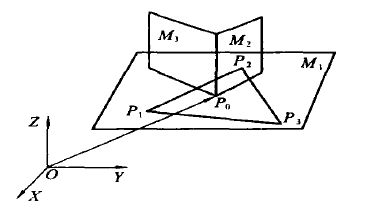如图所示，{O-XYZ}为机器人基座坐标系。P1、P2、P3为空间中任意不共线的三个点，其中P1 ( x 1 , y 1 , z 1 ) (x_{1}, y_{1}, z_{1}) 、P2 ( x 2 , y 2 , z 3 ) (x_{2}, y_{2}, z_{3}) 、P3 ( x 3 , y 3 , z 3 ) (x_{3}, y_{3}, z_{3}) 为三点在基座标系中的坐标。那么这三个点可以唯一确定一个外接圆，该园所在平面 M 1 M_{1} 的方程如下：
∣ x − x 3 y − y 3 z − z 3 x 1 − x 3 y 1 − y 3 z 1 − z 3 x 2 − x 3 y 2 − y 3 z 2 − z 3 ∣ = 0 （ 8 ） \left|\begin{array}{ccc}{x-x_{3}} & {y-y_{3}} & {z-z_{3}} \\ {x_{1}-x_{3}} & {y_{1}-y_{3}} & {z_{1}-z_{3}} \\ {x_{2}-x_{3}} & {y_{2}-y_{3}} & {z_{2}-z_{3}}\end{array}\right|=0 （8）
该外接圆方程的一般形式如下：
A 1 x + B 1 y + C 1 z + D 1 = 0 （ 9 ） A_{1} x+B_{1} y+C_{1} z+D_{1}=0（9）
其中，

A 1 = ( y 1 − y 3 ) ( z 2 − z 3 ) − ( y 2 − y 3 ) ( z 1 − z 3 ) A_{1} = (y_{1} - y_{3})(z_{2} - z_{3}) - (y_{2} - y_{3})(z_{1} - z_{3})

B 1 = ( x 2 − x 3 ) ( z 1 − z 3 ) − ( x 1 − x 3 ) ( z 2 − z 3 ) B_{1} = (x_{2}-x_{3})(z_{1} - z_{3}) - (x_{1} - x_{3})(z_{2} - z_{3})

C 1 = ( x 1 − x 3 ) ( y 2 − y 3 ) − ( x 2 − x 3 ) ( y 1 − y 3 ) C_{1} = (x_{1} - x_{3})(y_{2} - y_{3}) - (x_{2} - x_{3})(y_{1} - y_{3})

D 1 = − ( A 1 ⋅ x 3 + B 1 ⋅ y 3 + C 1 ⋅ z 3 ) D_{1} = -(A_{1}\cdot x_{3} + B_{1} \cdot y_{3} + C_{1} \cdot z_{3})

P1P2的垂直平分面M2的方程如下：
A 2 x + B 2 y + C 2 z + D 2 = 0 （ 10 ） A_{2} x+B_{2} y+C_{2} z+D_{2}=0（10）
其中，

A 2 = x 2 − x 1 A_{2} = x_{2} - x_{1}

B 2 = y 2 − y 1 B_{2} = y_{2} - y_{1}

C 2 = z 2 − z 1 C_{2} = z_{2} - z_{1}

D 2 = [ ( x 2 2 − x 1 2 ) + ( y 2 2 − y 1 2 ) + ( z 2 2 − z 1 2 ) ] / 2 D_{2} = \left[\left(x_{2}^{2}-x_{1}^{2}\right)+\left(y_{2}^{2}-y_{1}^{2}\right)+\left(z_{2}^{2}-z_{1}^{2}\right)\right] /2

P2P3的垂直平分面M3的方程如下：
A 3 x + B 3 y + C 3 z + D 3 = 0 （ 11 ） A_{3} x+B_{3} y+C_{3} z+D_{3}=0（11）
其中，

A 3 = x 3 − x 2 A_{3} = x_{3} - x_{2}

B 3 = y 3 − y 2 B_{3} = y_{3} - y_{2}

C 3 = z 3 − z 2 C_{3} = z_{3} - z_{2}

D 3 = − [ ( x 3 2 − x 2 2 ) + ( y 3 2 − y 2 2 ) + ( z 3 2 − z 2 2 ) ] / 2 D_{3} = -\left[\left(x_{3}^{2}-x_{2}^{2}\right)+\left(y_{3}^{2}-y_{2}^{2}\right)+\left(z_{3}^{2}-z_{2}^{2}\right)\right] /2

综上，联立M1、M2、M3三个平面的方程，可得到如下算式：
∣ A 1 B 1 C 1 A 2 B 2 C 2 A 3 B 3 C 3 ∣ ∣ x y z ∣ = ∣ − D 1 − D 2 − D 3 ∣ （ 12 ） \left|\begin{array}{lll}{A_{1}} & {B_{1}} & {C_{1}} \\ {A_{2}} & {B_{2}} & {C_{2}} \\ {A_{3}} & {B_{3}} & {C_{3}}\end{array}\right|\left|\begin{array}{l}{x} \\ {y} \\ {z}\end{array}\right|=\left|\begin{array}{c}{-D_{1}} \\ {-D_{2}} \\ {-D_{3}}\end{array}\right|（12）
公式（12）有且仅有唯一一组解，可解得三点外接圆圆心P0的位置坐标为 ( x 0 , y 0 , z 0 ) (x_{0}, y_{0}, z_{0}) ，根据圆心坐标可求得外接圆半径为
r = ( x 1 − x 0 ) 2 + ( y 1 − y 0 ) 2 + ( z 1 − z 0 ) 2 （ 13 ） r=\sqrt{\left(x_{1}-x_{0}\right)^{2}+\left(y_{1}-y_{0}\right)^{2}+\left(z_{1}-z_{0}\right)^{2}}（13）

#### 2.坐标变换

在以圆心P0为原点的基础上建立新的坐标系{P0-X0Y0Z0}。新坐标系中的Z0为平面M1的法矢量，从上文可得Z0轴的方向余弦为：
a x = A 1 / L , a y = B 1 / L ， a z = C 1 / L （ 14 ） a_{x}=A_{1} / L, \quad a_{y}=B_{1} / L， \quad a_{z}=C_{1} / L（14）
其中， L = ( A 1 2 + B 1 2 + C 1 2 ) 1 / 2 L=\left(A_{1}^{2}+B_{1}^{2}+C_{1}^{2}\right)^{1 / 2}

此处，规定新坐标系{P0-X0Y0Z0}的X0为P0P1的矢量方向，故可得X0轴的方向余弦如下：
n i = ( i 1 − i 0 ) ( x 1 − x 0 ) 2 + ( y 1 − y 0 ) 2 + ( z 1 − z 0 ) 2 （ 15 ） n_{i}=\frac{\left(i_{1}-i_{0}\right)}{\sqrt{\left(x_{1}-x_{0}\right)^{2}+\left(y_{1}-y_{0}\right)^{2}+\left(z_{1}-z_{0}\right)^{2}}}（15）
其中，i= x， y， z。

确定Z0和X0的方向后，根据右手定则即可确定Y0的方向，如下：
Y 1 = X 1 × Z 1 （ 16 ） \boldsymbol{Y}_{1}=\boldsymbol{X}_{1} \times \boldsymbol{Z}_{1}（16）
在得到新坐标系三个轴的方向余弦后，根据旋转矩阵的定义，可得到新坐标系{P0-X0Y0Z0}相对于基座标系{O-XYZ}的齐次变换矩阵
T = [ X 1 Y 1 Z 1 P 1 0 0 0 1 ] （ 17 ） T=\left[\begin{array}{cccc}{X_{1}} & {Y_{1}} & {Z_{1}} & {P_{1}} \\ {0} & {0} & {0} & {1}\end{array}\right]（17）
因此，可通过齐次变换矩阵T将基座标系内的空间圆弧变换到新坐标系内的平面圆弧，简化求解过程。

#### 3.转换为平面圆弧问题

根据齐次变换矩阵，P1、P2、P3在新坐标系下的坐标Q1、Q2、Q3可通过下面公式计算得到：
∣ Q i , 1 ∣ T = T − 1 ∣ P i , 1 ∣ T ( i = 1 , 2 , 3 ) （ 18 ） \left|Q_{i}, 1\right|^{\mathrm{T}}=T^{-1}\left|P_{i}, 1\right|^{\mathrm{T}}(i=1,2,3)（18）
在平面内进行圆弧插补，实际上就是对圆心角进行插补，因此核心就是求出圆弧的圆心角，同时还需要注意顺逆时针的问题。

#### 4.圆心角和插值点数N

由于atan2函数返回的是原点至点(x,y)的方位角，即与 x 轴的夹角。也可以理解为复数 x+yi 的辐角。返回值的单位为弧度。在数学坐标系中，结果为正表示从 X 轴逆时针旋转的角度，结果为负表示从 X 轴顺时针旋转的角度。此时为了判断圆弧是顺时针还是逆时针，需要将角度值转换成0~2 π \pi 的范围。

假设 ∠ P 1 P 0 P 2 \angle P_{1} P_{0} P_{2} P 0 P 2 P_{0}P_{2} P 0 X 0 P_{0}X_{0} 正向的夹角，有
∠ P 1 P 0 P 2 = atan ⁡ 2 ( P 2 y , P 2 x ) + n ∗ 2 π （ 19 ） \angle P_{1} P_{0} P_{2}=\operatorname{atan} 2\left(P_{2 y}, P_{2 x}\right)+n * 2 \pi（19）
其中 P 2 y 、 P 2 x P_{2y}、P_{2x} 是P2在新坐标系下的坐标值。此时存在如下两种情况：

1. atan ⁡ 2 ( P 2 y , P 2 x ) < 0 \operatorname{atan} 2\left(P_{2 y}, P_{2 x}\right)<0 时， n = 1 n=1
2. 否则， n = 0 n=0

同理，假设 ∠ P 1 P 0 P 3 \angle P_{1} P_{0}P_{3} P 0 P 3 P_{0}P_{3} P 0 X 0 P_{0}X_{0} 正向的夹角，有
∠ P 1 P 0 P 3 = atan ⁡ 2 ( P 3 y , P 3 x ) + n ∗ 2 π （ 20 ） \angle P_{1} P_{0} P_{3}=\operatorname{atan} 2\left(P_{3 y}, P_{3 x}\right)+n * 2 \pi（20）
其中 P 3 y 、 P 3 x P_{3y}、P_{3x} 是P2在新坐标系下的坐标值。此时存在如下两种情况：

1. atan ⁡ 2 ( P 3 y , P 3 x ) < 0 \operatorname{atan} 2\left(P_{3 y}, P_{3 x}\right)<0 时， n = 1 n=1
2. 否则， n = 0 n=0

通过比较 ∠ P 1 P 0 P 2 \angle P_{1} P_{0} P_{2} ∠ P 1 P 0 P 3 \angle P_{1} P_{0} P_{3} 的大小可以确定圆弧的方向，同时可以求出圆弧圆心角 Ω \Omega

1. ∠ P 1 P 0 P 2 < ∠ P 1 P 0 P 3 \angle P_{1} P_{0} P_{2}<\angle P_{1} P_{0} P_{3} 时，圆弧为逆时针，圆心角 Ω = ∠ P 1 P 0 P 3 \Omega=\angle P_{1} P_{0} P_{3} ;
2. ∠ P 1 P 0 P 2 ≥ ∠ P 1 P 0 P 3 \angle P_{1} P_{0} P_{2} \geq \angle P_{1} P_{0} P_{3} 时， Ω = 2 π − ∠ P 1 P 0 P 3 \Omega=2\pi - \angle P_{1} P_{0} P_{3} ;

对应基于抛物线过渡（梯形加减速）的空间圆弧插补而言，需要初始化的运动参数有机械臂末端角速度 ω s \omega_{s} 和角加速度a，需要计算的插值参数为圆心角 Ω \Omega 和插值点数N。上文已经求得圆心角，插值点数计算公式如下：
N = P n Ω ω s （ 21 ） \mathrm{N}=P_{n} \frac{\Omega}{\omega_{s}}（21）

## 归一化因子 λ \lambda 的求解

预备知识

机器人学回炉重造（6）：关节空间规划方法——梯形加减速（与抛物线拟合的线性函数）、S型曲线规划

设机械臂在匀速运动时的线速度为 v s v_{s} ，抛物线段的加减速度均为 a a ，可以计算出加速阶段和减速阶段的时间和位移为
T 1 = V s a （ 22 ） T_{1}=\frac{V_{s}}{a}（22）

S 1 = 1 2 a T 1 2 （ 23 ） S_{1}=\frac{1}{2} a T_{1}^{2}（23）

设机械臂末端运动的总位移为 S e S_{e} ，总时间为：
T e = 2 T 1 + s e − 2 S 1 V s （ 24 ） T_{e}=2 T_{1}+\frac{s_{e}-2 S_{1}}{V_{s}}（24）
将位移、速度和加速度进行归一化处理得到上述计算量的归一化参数：
S 1 λ = s 1 s e （ 25 ） S_{1 \lambda}=\frac{s_{1}}{s_{e}}（25）

T 1 λ = T 1 T e （ 26 ） T_{1 \lambda}=\frac{T_{1}}{T_{e}}（26）

T 2 λ = 1 − T 1 λ （ 27 ） T_{2 \lambda}=1-T_{1 \lambda}（27）

a λ = 2 s 1 λ T 1 λ 2 （ 28 ） a_{\lambda}=\frac{2 s_{1 \lambda}}{T_{1 \lambda}^{2}}（28）

结合梯形加减速的位移公式，可以推导出归一化因子 λ \lambda 的计算公式如下：
λ = { 1 2 a λ t 2 ( 0 ≤ t ≤ T 1 λ ) 1 2 a λ T 1 λ 2 + a λ T 1 λ ( t − T 1 λ ) ( T 1 λ < t ≤ T 2 λ ) 1 2 a λ T 1 λ 2 + a λ T 1 λ ( t − T 1 λ ) − 1 2 a λ ( t − T 2 λ ) 2 ( T 2 λ < t ≤ 1 ) （ 29 ） \lambda=\left\{\begin{array}{cc}{\frac{1}{2} a_{\lambda} t^{2}} & {\left(0 \leq t \leq T_{1 \lambda}\right)} \\ {\frac{1}{2} a_{\lambda} T_{1 \lambda}^{2}+a_{\lambda} T_{1 \lambda}\left(t-T_{1 \lambda}\right)} & {\left(T_{1 \lambda}<t \leq T_{2 \lambda}\right)} \\ {\frac{1}{2} a_{\lambda} T_{1 \lambda}^{2}+a_{\lambda} T_{1 \lambda}\left(t-T_{1 \lambda}\right)-\frac{1}{2} a_{\lambda}\left(t-T_{2 \lambda}\right)^{2}} & {\left(T_{2 \lambda}<t \leq 1\right)}\end{array}\right.（29）
式中，t表示时间， t = i / N ( 0 < = t < = 1 ) t=i/N(0<=t<=1) ，其中N表示总的插值点数，i = 1、2、3、……、N-1、N。每个插值点的时间值，都有一个 λ \lambda 与之对应，结合公式（1）（2）（3）可以得到各个插值点位置坐标向量和RPY角度向量，最后就是解逆运动学方程，得到每个插值点对应的关节变量，生成轨迹。

## Matlab实现代码

预备知识：

五自由度机械臂正逆运动学算法（Ｃ语言+Matlab）

模型是我买的五自由度机械臂，之前已经推导过逆运动学的解析解，这里直接拿来用。

先是预备程序：

• 高斯列主元消去法，解圆心方程；
• 五自由度逆运动学解析解推导（前面博客有）；
• 归一化因子推导。
%% 高斯列主元消去法
function x = Gauss_lie(n, A, b)
for k = 1: n-1
a_max = abs(A(k, k));
cnt = k;
for i = k: n
if (abs(A(i, k)) > a_max)
a_max = abs(A(i, k));
cnt = i; % 确定下标i
end
end
if (A(cnt, k) == 0)
fprintf('Gauss_lie: no unique solution\n');
return;
end
% 换行
if (cnt ~= k)
t = 0; s = 0;
for j = k: n
t = A(k, j);
A(k, j) = A(cnt, j);
A(cnt, j) = t;
s = b(cnt);
b(cnt) = b(k);
b(k) = s;
end
end
% step 5
for i = k+1: n
L(i) = A(i, k) / A(k, k);
for j = k+1: n
A(i, j) = A(i, j) - L(i)*A(k, j);
end
b(i) = b(i) - L(i)*b(k);
end
end
for i = 1: n
if (A(i, i) == 0)
fprintf('Gauss_lie no unique solution\n');
return;
end
end
% 回代
x(n) = b(n) / A(n, n);
for i = n-1: -1: 1
sum_a = 0;
for j = i+1: n
sum_a = sum_a + A(i, j)*x(j);
end
x(i) = (b(i) - sum_a) / A(i, i);
end

end

% 归一化处理
% 梯形加减速曲线
% 输入参数：机械臂末端运动总位移（角度）pos
%          机械臂末端线速度（角速度）vel
%          加速度减速度accl（设定加减速段accl相同）
%          插值点数N
function lambda = Normalization(pos, vel, accl, N)
% 加减速段的时间和位移
T1 = vel / accl;
S1 = (1/2) * accl * T1^2;
% 总时间
Te = 2*T1 + (pos - 2*S1) / vel;
% 归一化处理
S1_ = S1 / pos;
T1_ = T1 / Te;
T2_ = 1 - T1_;
accl_ = 2*S1_ / T1_^2;
% lambda求解公式
for i = 0: N
t = i / N;
if (t >= 0 && t <= T1_)
lambda(i+1) = (1/2) * accl_ * t^2;
elseif (t > T1_ && t <= T2_)
lambda(i+1) = (1/2)*accl_*T1_^2 + accl_*T1_*(t - T1_);
elseif (t > T2_ && t <= 1)
lambda(i+1) = (1/2)*accl_*T1_^2 + accl_*T1_*(T2_ - T1_) + (1/2)*accl_*power(t - T2_, 2);
end
end

end


核心程序：

% 空间直线位置插补与RPY角姿态插补 + 梯形加减速归一化处理
% 参数：起点S位置， 终点D位置, 末端线速度vs， 加减速度a
%      起点S的RPY角、终点D的RPY角
% 返回值：插值点（不包括起点S和终点D）
function [x y z alp beta gama N] = SpaceLine(S, D, S_, D_, vs, a)
x1 = S(1); y1 = S(2); z1 = S(3);
x2 = D(1); y2 = D(2); z2 = D(3);
alp1 = S_(1); beta1 = S_(2); gama1 = S_(3);
alp2 = D_(1); beta2 = D_(2); gama2 = D_(3);
P = 1; % 插值参数，增加插值点数，避免过小
% 总位移S
s = sqrt(power(x2 - x1, 2) + power(y2 - y1, 2) + power(z2 - z1, 2))
% 插值点数N
N = ceil(P*s / vs)
% 求归一化参数
% function lambda = Normalization(pos, vel, accl, N)
lambda = Normalization(s, vs, a, N)
delta_x = x2 - x1;
delta_y = y2 - y1;
delta_z = z2 - z1;
delta_alp = alp2 - alp1;
delta_beta = beta2 - beta1;
delta_gama = gama2 - gama1;
for i = 1: N+1
x(i) = x1 + delta_x*lambda(i);
y(i) = y1 + delta_y*lambda(i);
z(i) = z1 + delta_z*lambda(i);
alp(i) = alp1 + delta_alp*lambda(i);
beta(i) = beta1 + delta_beta*lambda(i);
gama(i) = gama1 + delta_gama*lambda(i);
end
end

% 空间圆弧位置插补与RPY角姿态插补 + 梯形加减速归一化处理
% 参数： 起点S位置和RPY角, 中间点M位置, 终点D位置和RPY角，末端角速度，角加减速度
% 方便起见，角速度和角加速度均为角度制
function [x y z alp beta gama N] = SpaceCircle(S, M, D, S_, D_, ws, a)
x1 = S(1); x2 = M(1); x3 = D(1);
y1 = S(2); y2 = M(2); y3 = D(2);
z1 = S(3); z2 = M(3); z3 = D(3);
alp1 = S_(1); beta1 = S_(2); gama1 = S_(3);
alp2 = D_(1); beta2 = D_(2); gama2 = D_(3);

A1 = (y1 - y3)*(z2 - z3) - (y2 - y3)*(z1 - z3);
B1 = (x2 - x3)*(z1 - z3) - (x1 - x3)*(z2 - z3);
C1 = (x1 - x3)*(y2 - y3) - (x2 - x3)*(y1 - y3);
D1 = -(A1*x3 + B1*y3 + C1*z3);

A2 = x2 - x1;
B2 = y2 - y1;
C2 = z2 - z1;
D2 = -((x2^2 - x1^2) + (y2^2 - y1^2) + (z2^2 - z1^2)) / 2;

A3 = x3 - x2;
B3 = y3 - y2;
C3 = z3 - z2;
D3 = -((x3^2 - x2^2) + (y3^2 - y2^2) + (z3^2 - z2^2)) / 2;
A = [A1, B1, C1; A2, B2, C2; A3, B3, C3]
b = [-D1, -D2, -D3]'
% 圆心
C = Gauss_lie(3, A, b)
x0 = C(1); y0 = C(2); z0 = C(3);
plot3(x0, y0, z0, 'bo')
hold on
% 外接圆半径
r = sqrt(power(x1 - x0, 2) + power(y1 - y0, 2) + power(z1 - z0, 2));
% 新坐标系Z0的方向余弦
L = sqrt(A1^2 + B1^2 + C1^2);
ax = A1 / L; ay = B1 / L; az = C1 / L;
% 新坐标系X0的方向余弦
nx = (x1 - x0) / r;
ny = (y1 - y0) / r;
nz = (z1 - z0) / r;
% 新坐标系Y0的方向余弦
o = cross([ax, ay, az], [nx, ny, nz]);
ox = o(1);
oy = o(2);
oz = o(3);
% 相对于基座标系{O-XYZ}， 新坐标系{C-X0Y0Z0}的坐标变换矩阵
T = [nx ox ax x0;
ny oy ay y0;
nz oz az z0;
0  0  0  1]
T_ni = T^-1
% 求在新坐标系{C-X0Y0Z0}下S、M和D的坐标
S_ = (T^-1)*[S'; 1]
M_ = (T^-1)*[M'; 1]
D_ = (T^-1)*[D'; 1]
x1_ = S_(1) , y1_ = S_(2), z1_ = S_(3)
x2_ = M_(1), y2_ = M_(2), z2_ = M_(3)
x3_ = D_(1), y3_ = D_(2), z3_ = D_(3)
% 判断圆弧是顺时针还是逆时针，并求解圆心角
if (atan2(y2_, x2_) < 0)
angle_SOM = atan2(y2_, x2_) + 2*pi;
else
angle_SOM = atan2(y2_, x2_);
end
if (atan2(y3_, x3_) < 0)
angle_SOD = atan2(y3_, x3_) + 2*pi;
else
angle_SOD = atan2(y3_, x3_);
end
% 逆时针
if (angle_SOM < angle_SOD)
flag = 1;
theta = angle_SOD % 圆心角
end
% 顺时针
if (angle_SOM >= angle_SOD)
flag = -1;
theta = 2*pi - angle_SOD % 圆心角
end
% 插补点数N
P = 2; %插补参数，增加插值点数，避免过小
ws = ws*pi / 180; % 角度换成弧度
a = a*pi / 180;
N = P*theta / ws;
% 求归一化参数
lambda = Normalization(theta, ws, a, N);

% 插补原理: 在新平面上进行插补（简化）
% 在新坐标系下z1_,z2_,z3_均为0，即外接圆在新坐标系的XOY平面内
% 此时转化为平面圆弧插补问题
delta_ang = theta;
delta_alp = alp2 - alp1
delta_beta = beta2 - beta1;
delta_gama = gama2 - gama1;
for i = 1: N+1
x_(i) = flag * r * cos(lambda(i)*delta_ang);
y_(i) = flag * r * sin(lambda(i)*delta_ang);
P = T*[x_(i); y_(i); 0; 1];
x(i) = P(1);
y(i) = P(2);
z(i) = P(3);
alp(i) = alp1 + delta_alp*lambda(i);
beta(i) = beta1 + delta_beta*lambda(i);
gama(i) = gama1 + delta_gama*lambda(i);
end
% % figure(1)
% % plot(x_, y_)
% 插补原理： 在原圆弧上进行插补
% 圆弧上任一点处沿前进方向的切向量
% x(1) = x1; y(1) = y1; z(1) = z1;
% for i = 1: N+1
%     m(i) = flag*(ay*(z(i) - z0) - az*(y(i) - y0));
%     n(i) = flag*(az*(x(i) - x0) - ax*(z(i) - z0));
%     l(i) = flag*(ax*(y(i) - y0) - ay*(x(i) - x0));
%     delta_s = delta_ang * r;
%     E = delta_s / (r*sqrt(ax^2 + ay^2 + az^2));
%     G = r / sqrt(r^2 + delta_s^2);
%     x(i+1) = x0 + G*(x(i) + E*m(i) - x0);
%     y(i+1) = y0 + G*(y(i) + E*n(i) - y0);
%     z(i+1) = z0 + G*(z(i) + E*l(i) - z0);
% end

end


测试主程序

% Standard DH
% five_dof robot
% 在关节4和关节5之间增加一个虚拟关节，便于逆运动学计算
clear;
clc;
th(1) = 0; d(1) = 0; a(1) = 0; alp(1) = pi/2;
th(2) = 0; d(2) = 0; a(2) = 1.04;alp(2) = 0;
th(3) = 0; d(3) = 0; a(3) = 0.96; alp(3) = 0;
th(4) = 0; d(4) = 0; a(4) = 0; alp(4) = 0;
th(5) = pi/2; d(5) = 0; a(5) = 0; alp(5) = pi/2;
th(6) = 0; d(6) = 0; a(6) = 0; alp(6) = 0;
th(7) = 0; d(7) = 1.63; a(7) = 0.28; alp(7) = 0;
% DH parameters  th     d    a    alpha  sigma
L1 = Link([th(1), d(1), a(1), alp(1), 0]);
L2 = Link([th(2), d(2), a(2), alp(2), 0]);
L3 = Link([th(3), d(3), a(3), alp(3), 0]);
L4 = Link([th(4), d(4), a(4), alp(4), 0]);
L5 = Link([th(5), d(5), a(5), alp(5), 0]);
L6 = Link([th(6), d(6), a(6), alp(6), 0]);
L7 = Link([th(7), d(7), a(7), alp(7), 0]);
robot = SerialLink([L1, L2, L3, L4, L5, L6, L7]);
robot.name='MyRobot-5-dof';
robot.display()

% 起点关节角[0 90 30 60 90 0 0]*pi/180
% 终点关节角[0 90 60 60 90 0 0]*pi/180
theta_S = [0 120 100 40 90 0 0]*pi/180;
theta_M = [60 100 100 30 90 0 0]*pi/180;
theta_D = [100 120 100 40 90 0 0]*pi/180;
% robot.teach();
% robot.plot(theta_D);
% hold on
T_S = robot.fkine(theta_S)    %起点末端执行器位姿
T_M = robot.fkine(theta_M)
T_D = robot.fkine(theta_D)
% ik_T = five_dof_ikine(T_D)
[S_RPY(1) S_RPY(2) S_RPY(3)] = RPY_angle(T_S); % 起点对应的RPY角
[D_RPY(1) D_RPY(2) D_RPY(3)] = RPY_angle(T_D); % 终点对应的RPY角
S = T_S(1: 3, 4); % 起点对应的位置坐标
M = T_M(1: 3, 4);
D = T_D(1: 3, 4); % 终点对应的位置坐标
vs = 0.06; a = 0.02; % 直线插补速度参数
ws = 5; a = 2.5; % 空间圆弧插补速度参数
% [x y z alp beta gama N] = SpaceLine(S, D, S_RPY, D_RPY, vs, a); % 直线插补，得到插值点（不包括起点和终点）
[x y z alp beta gama N] = SpaceCircle(S', M', D', S_RPY, D_RPY, ws, a);
plot3(x, y, z)
hold on
th1(1) = theta_S(1); th2(1) = theta_S(2); th3(1) = theta_S(3);
th4(1) = theta_S(4); th5(1) = theta_S(5); th6(1) = theta_S(6); th7(1) = theta_S(7);
% t = [0 0 0 0 0];
T = {1, N};
for i = 1: N+1
R = RPY_rot(alp(i), beta(i), gama(i));
R(1, 1:3);
R(2, 1:3);
R(3, 1:3);
T{i} = [R(1, 1:3), x(i);
R(2, 1:3), y(i);
R(3, 1:3), z(i);
0 0 0 1];
theta = five_dof_ikine(T{i});
th = theta(2, 1:5); % 简单取1行逆解
th1(i+1) = th(1);
th2(i+1) = th(2);
th3(i+1) = th(3);
th4(i+1) = th(4);
th5(i+1) = pi/2;
th6(i+1) = th(5);
th7(i+1) = 0;
end
for i = 1: N+1
T_ = robot.fkine([th1(i), th2(i), th3(i), th4(i), th5(i), th6(i), th7(i)]);
traj = T_(1: 3, 4);
a(i) = traj(1);
b(i) = traj(2);
c(i) = traj(3);
plot3(traj(1), traj(2), traj(3), 'r*');
hold on
robot.plot([th1(i), th2(i), th3(i), th4(i), th5(i), th6(i), th7(i)])
end


## 参考文献

杨淞. 一种六自由度机械臂的运动控制系统设计[D].上海交通大学,2014.

陈国良,黄心汉,王敏.机械手圆周运动的轨迹规划与实现[J].华中科技大学学报(自然科学版),2005(11):69-72.

卓扬娃,白晓灿,陈永明.机器人的三种规则曲线插补算法[J].装备制造技术,2009(11):27-29.

林威,江五讲.工业机器人笛卡尔空间轨迹规划[J].机械工程与自动化,2014(05):141-143.

Chen Z, Wang H, Lu X, et al. Kinematics analysis and application of 5-DOF manipulator with special joint[C]//2017 Chinese Automation Congress (CAC). IEEE, 2017: 7421-7426.

展开全文机器人学
• 本发明属于运动控制领域，具体涉及一种基于移动平均算法的S曲线加减速控制方法。...一般常用的减速算法包括直线加减速，指数型加减速S曲线加减速。前两种虽然算法简单，但是不能够进行柔性加工，...
• 曲线加减速规划是否存在匀速段取决于速度达到给定的指令速度口时，加速段和减速段位移之和s'是否小于待插补位移s． 加速及减速过程中是否存在匀加速段或匀减速段则由vs,ve,v,a,j决定。 规划后的S曲线可能出现下图中...算法 二分法
• 对于连续多段路径，传统方法是将多段路径细分，然后对每段路径采用首尾速度为0的加减速算法（S型曲线或梯形曲线），这就带来了频繁启停的问题，容易对机械臂造成冲击，同时运行时间较长。 下面我把前面博客中提到的...Matlab
• 1、西门子S7_1500实现判断当前位置与目标位置的关系，基于sinxsinx函数自动生成S型曲线轮廓，并使用插补对轮廓进行密集插值，保证曲线的平滑度。 2、由于 SinxSinx 曲线具有的一阶二阶连续性，速度和加加速度都...
• {lim}$：最大速度$q_0$：初始行程$q_1$：终点行程$v_0$：初始速度$v_1$：终点速度$a_0$：初始速度$a_1\$：终点速度 由此可知，S型速度规划的特定是能够使得机械臂平滑加速到最大运行速度，然后又平滑地减速，而且...
• 运动规划指的是运动插补，在起始点和终止点之间插入中间点序列，实现沿着轨迹的平稳运动。运动控制包含路径规划(path planning)和轨迹规划，路径规划是规划位置，在起终点之间经过的路径点，轨迹规划是规划时间，将...matlab
• S速度规划代码OpenRobotSL/TrajectoryPlanningS速度规划代码https://github.com/OpenRobotSL/TrajectoryPlanning​github.com基于S型曲线的连续多段曲线插补平滑过渡的规划算法（Matlab）_xuuyann-CSDN博客​blog....
• 步进电机三轴联动的快速加减速算法研究步进电机三轴联动的快速加减速算法研究1引言步进电机具有快速启停能力强、精度高、转速容易控制的特点。但是，步进电动机应避免转动速率的突变，而且从停止到开始转动有一个较...
• 已知线段长度sss，起点速度v0v_0v0​，利用速度S形算法（滤波方式）计算能达到的最大终点速度和最小终点速度。其中，系统最大速度为vmv_mvm​，系统最大速度为ama_mam​，系统最大加加速度为JmJ_mJm​，插补周期...算法
• 对于插补技术的理解与认识 1 插补及其算法概述 1.1 什么是插补 CNC系统之所以能够控制刀具中心相对于工件以一定的速度和轨迹运动，切削出工件的轮廓，是由于CNC具有插补功能。所谓的插补就是根据零件轮廓尺寸，结合...矩阵
• S加减速–5段 为了减小机器人加速过程的冲击，这里梳理一下S加减速相关知识。 1. S加减速曲线 计算公式： 加加速度 j(t)={J0≤t≤t1−Jt1≤t≤t20t2≤t≤t3−Jt3≤t≤t4Jt4≤t≤t5 j(t)=\begin{cases} J &...算法 动态规划
• 一种连续小线段高速插补算法,前瞻线段插补算法,插补算法,圆弧插补算法,c语言圆弧插补算法,直线插补算法,三轴直线插补算法,螺旋插补算法,plc圆弧插补算法实例,plc圆弧插补算法( )第 32 卷 第 4 期 南京理工大学学报 ...
• 对于连续多段路径，传统方法是将多段路径细分，然后对每段路径采用首尾速度为0的加减速算法（S型曲线或梯形曲线），这就带来了频繁启停的问题，容易对机械臂造成冲击，同时运行时间较长。 下面我把前面博客中提到的...
• 知乎话题：多轴插补为什么普遍使用梯形速度曲线？ 链接：https://www.zhihu.com/people/yu-tian-qi-76/activities 1-ICRA2014上 Joonyoung Kim分享了Trajectory Planning for Robots: The Challenges of ...
• 型曲线加减速前瞻控制算法，根据加工路径的型值点的实际情况，确定每一型值点处的最大衔接速度，采用S型曲线加减速控 制，实现各路径段之间进给速度的快速衔接，从而达到高速高精的运动控制目的。运动控制
•  S型速度曲线  为了使速度连续，可对梯形速度规划中的速度曲线进行修改，使速度曲线变为连续的二次曲线(a)或者梯形曲线(b)，如下图...
• 采用STM系列开发板，实现智能运动控制的插补运算、加减速控制，要求结合开发板模块，使用相关算法，可靠实现电机的控制 最后的结果应该是能通过stm32控制电机，实现目标按s型轨迹运动和加减速，正反转
• 1 引言 步进电机具有快速启停能力强、精度高、转速容易控制的特点。但是，步进电动机应避免转动速率的突变，而且从停止到开始转动有一个较低的起动频率，随后才可以平缓地过渡到较高...目前国内外步进电机加减速控制曲
• ## GRBL源码解析

千次阅读 2020-05-16 22:12:26
重点还是关注圆弧拆分线段、转角速度优化、线段梯形加减速插补和自动归位的实现方法。 一、串口接收 串口配置成中断接收和中断发送模式，并创建了串口接收环形队列和串口发送环形队列，中断接收的数据存放在串口接收......